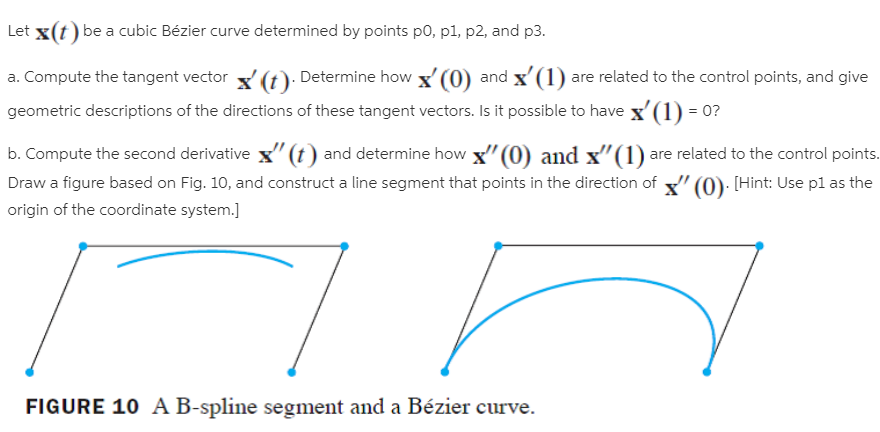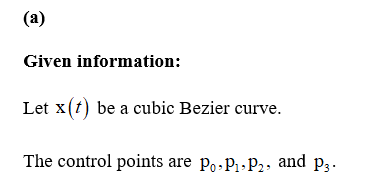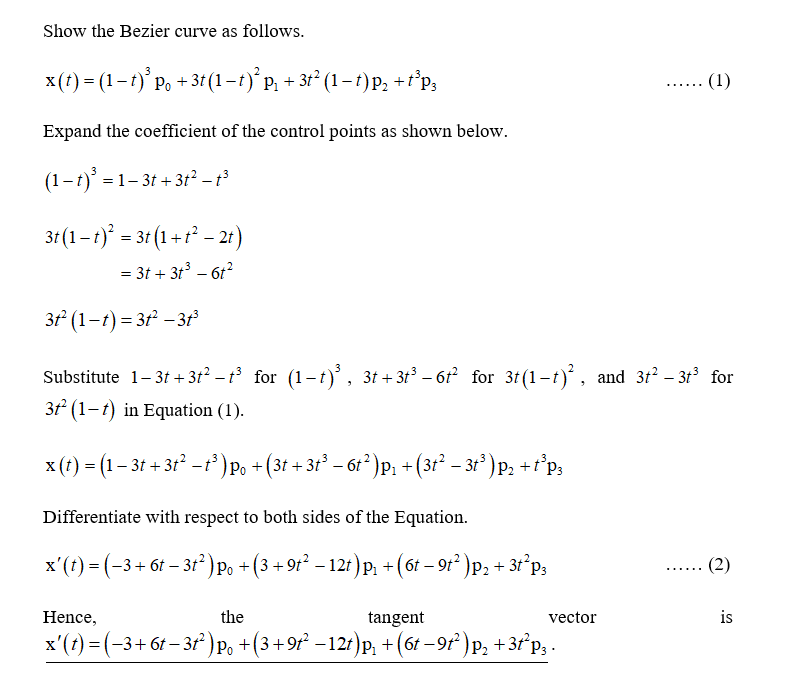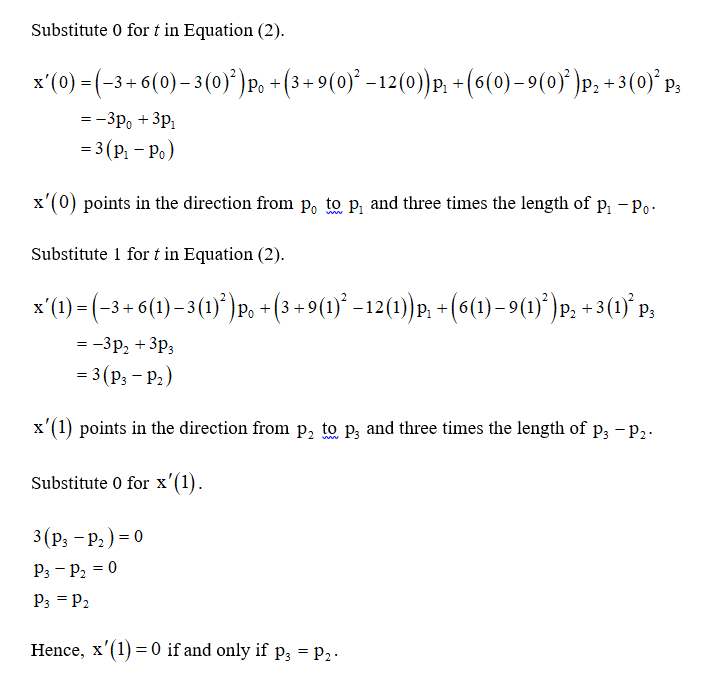# Let x(t) be a cubic Bézier curve determined by points po, p1, p2, and p3.a. Compute the tangent vector (t). Determine how x' (0) and x'(1) are related to the control points, and givegeometric descriptions of the directions of these tangent vectors. Is it possible to have x'(1) = 0?b. Compute the second derivative x" (t) and determine how x"(0) and x"(1) are related to the control points.Draw a figure based on Fig. 10, and construct a line segment that points in the direction of " (0): [Hint: Use pl as theorigin of the coordinate system.]FIGURE 10 A B-spline segment and a Bézier curve.

Question
1 viewshelp_outlineImage TranscriptioncloseLet x(t) be a cubic Bézier curve determined by points po, p1, p2, and p3. a. Compute the tangent vector (t). Determine how x' (0) and x'(1) are related to the control points, and give geometric descriptions of the directions of these tangent vectors. Is it possible to have x'(1) = 0? b. Compute the second derivative x" (t) and determine how x"(0) and x"(1) are related to the control points. Draw a figure based on Fig. 10, and construct a line segment that points in the direction of " (0): [Hint: Use pl as the origin of the coordinate system.] FIGURE 10 A B-spline segment and a Bézier curve. fullscreen
check_circle

Step 1Step 2Step 3...

### Want to see the full answer?

See Solution

#### Want to see this answer and more?

Solutions are written by subject experts who are available 24/7. Questions are typically answered within 1 hour.*

See Solution
*Response times may vary by subject and question.
Tagged in